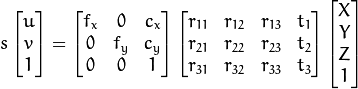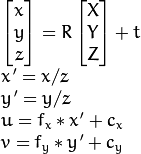# Solvepnp 5 Methods In Opencv3

#### Before Begin

SolvePnP , Finds an object pose from 3D-2D point correspondences., which is the method that rebuild 3D position from 2D picture.

We DO NOT Care about the how to use SolvePnP, we try to understand the inner meaning of SolvePnP, and the 5 methods.

#### SolvePnP Methods and Paper table:

SolvePnP MethodsReference Paperyear
SOLVEPNP_ITERATIVEOriginal based on Levenberg-Marquardt optimization
SOLVEPNP_EPNPEPnP: Efficient Perspective-n-Point Camera Pose Estimation2008
SOLVEPNP_P3PComplete Solution Classification for the Perspective-Three-Point Problem2003
SOLVEPNP_DLSA Direct Least-Squares (DLS) Method for PnP2011
SOLVEPNP_UPNPExhaustive Linearization for Robust Camera Pose and Focal Length Estimation2013

Methods for solving a PnP problem:

• SOLVEPNP_ITERATIVE Iterative method is based on Levenberg-Marquardt optimization. In this case the function finds such a pose that minimizes reprojection error, that is the sum of squared distances between the observed projections imagePoints and the projected (using projectPoints ) objectPoints .
• SOLVEPNP_P3P Method is based on the paper of X.S. Gao, X.-R. Hou, J. Tang, H.-F. Chang “Complete Solution Classification for the Perspective-Three-Point Problem”. In this case the function requires exactly four object and image points.
• SOLVEPNP_EPNP Method has been introduced by F.Moreno-Noguer, V.Lepetit and P.Fua in the paper “EPnP: Efficient Perspective-n-Point Camera Pose Estimation”.
• SOLVEPNP_DLS Method is based on the paper of Joel A. Hesch and Stergios I. Roumeliotis. “A Direct Least-Squares (DLS) Method for PnP”.
• SOLVEPNP_UPNP Method is based on the paper of A.Penate-Sanchez, J.Andrade-Cetto, F.Moreno-Noguer. “Exhaustive Linearization for Robust Camera Pose and Focal Length Estimation”. In this case the function also estimates the parameters \f$f_x\f$ and \f$f_y\f$ assuming that both have the same value. Then the cameraMatrix is updated with the estimated focal length.

### 1. SOLVEPNP_ITERATIVE

Accroding to solvepnp.cpp:

else if (flags == SOLVEPNP_ITERATIVE)
{
CvMat c_objectPoints = opoints, c_imagePoints = ipoints;
CvMat c_cameraMatrix = cameraMatrix, c_distCoeffs = distCoeffs;
CvMat c_rvec = rvec, c_tvec = tvec;
cvFindExtrinsicCameraParams2(&c_objectPoints, &c_imagePoints, &c_cameraMatrix,
c_distCoeffs.rows*c_distCoeffs.cols ? &c_distCoeffs : 0,
&c_rvec, &c_tvec, useExtrinsicGuess );
result = true;
}


Which meanings this Method use the function cvFindExtrinsicCameraParams2 in calibration.cpp.

In this moment, should reference offical calib3d. In Detailed Desciption, focus on the perspective transformation.• (X, Y, Z) are the coordinates of a 3D point in the world coordinate space
• (R t) is the Camera’s Rotation and Position, which is what we need to know
• fx, fy, cx, cy is the Camera’s intrinsic
• s is Focal length
• u v are the coordinates of the projection point in pixels(picture)

or can use this table:In cvFindExtrinsicCameraParams2, you can get Camera extrinsic from intrinsic, camera intrinsic matrix and distortion coefficients.

Camera extrinsic is Camera’s Postion and Rotation, in the other word, if the Camera is static, then can get the Object’s Position and Rotation, but the output is reversed.

#### How to Calc cvFindExtrinsicCameraParams2

Back to cvFindExtrinsicCameraParams2, this function use DLT(Direct Linear Transform) algorithm solve it, and refine extrinsic parameters using Levenberg-Marquardt algorithm.Then, use Gaussion-Newton minimize the reprojection error.

### 2. SOLVEPNP_P3P

First, we have to know, the P, A, B, C must be Non-coplanar.use the law of cosines, we can know:Final, Fomulation(13), x can get 4 possible answer. So, use the 4th point to determind the best answer.### 3. SOLVEPNP_EPNP

Reference:

Key Word: SVD(Singular Value Decomposition)

#### 3.1 Mx = 0

First, you should understand that every point can be present by four base point in 3D space. In theory, the control points(four base points) can be chosen arbitrarily, However, in practice, found that the stability of the paper’s method is increased by taking the centroid of the reference points as one, and to select the rest in such a way that they form a basis aligned with the principal directions.

First, look at this basic fomulation: P^w meaning points in world coordinate, meanwhile P^c meaning points in camera coordinate. P^c is what we determind first, so every point can be present by four base point.

in this case,

is matrix multiplication, Present the object point transform from World coordinate to Camera coordinate, meanings the Object position in Camera view, and this can be present by four base points.

so,

and, from the last row, you can find

so, this matrix can be,

so, every point have two formulation, so, seems like,

[M] is [2n*12] Matrix, [X] is [12*1] (n meanings the number of Object Points, 12-vector unknow is these four basic points’ position in Camera View.)

Then, the Question is how to min the Mx. Simple say, The least squares solution is the eigenvector corresponding to the smallest singular value of M ^ TM (最小二乘解就是 M^TM的最小的奇异值所对应的特征向量)。This can get X, meanings C_j^w, base points in Camera coordinate.

Because of the noise, we can not just get the x from Mx=0, Although we can calculate the x by Least Squares, which can get answer by M^TMx = M^T0, but we can not only focus the min eigenvector in SVD, we need the 2nd min eigenvector and more .

So we need do SVD from A^TA, get the Vj(j is the last 4 column)

Reference: SVD-PPT-Chinese

#### 3.2 L * beta=P

Focus on X, we know that the solution is smallest singular value, but with the noise, we have to consider penultimate sigular value and others, so consider the follow formulation.

With the paper, we know that the N can be 1,2,3 and 4. When f is small, meanings we choose a small focal length, M^TM have only one zero eigenvalue, however, as the focal length increases and camera becomes closer to being orthograhic, all four smallest eigenvalues approach zero, so the N can be 4. Furthermore, if the correspondences are noisy, the smallest of them will be tiny but not strictly equal to zero.

if We do not have noise, we can know the N = 1, and the X is the vector which the eigenvalue is zero. but in natural, the noise and the Object points can not be precise, so we have to make N to be 3 or 4.

so we have to solve beta_k. The stratage is whether in World coordinate or Camera coordinate, the length between two points is static, so,#### 3.3 GN(Gaussion Newton iteration)

Iteration beta, min the cost

![Error(\beta )=\sum {(i,j) i < j}(\Vert c_i^c - c_j^c\Vert^2-\Vert c_i^w - c_j^w\Vert^2)](https://latex.codecogs.com/png.latex?%5Cinline%20Error%28%5Cbeta%20%29%3D%5Csum%20%7B%28i%2Cj%29%20i%20%3C%20j%7D%28%5CVert%20c_i%5Ec%20-%20c_j%5Ec%5CVert%5E2-%5CVert%20c_i%5Ew%20-%20c_j%5Ew%5CVert%5E2%29)

have to focus on Jacobi iteration.in Newton method:

so, in our Error:

in Picture, this formulation is :

like this:#### 3.4 Recover 3d points in camera coordinate

After GN, we can get the best _beta, so we can get the best P^c , with P^w, we can get R and t.

![p_i^c=\sum {j=1}^4\alpha _{ij}c_j^c](https://latex.codecogs.com/png.latex?%5Cinline%20p_i%5Ec%3D%5Csum%20%7Bj%3D1%7D%5E4%5Calpha%20_%7Bij%7Dc_j%5Ec)

#### 3.5 Conclusion

we can use EPnP get the R and t, then use SOLVEPNP_ITERATIVE GN do the last optimize.todo

### 5. SOLVEPNP_UPNP

UPnp is similar with EPnP, but UPnP do not know the matrix of intrinsic parameters, eg.(cx,cy) is a principal point that is usually at the image center ,fx,fy are the focal lengths expressed in pixel units.

in general, caculate the

in this case, N can be 1 or 2, sometimes, the with little Object point and too much noise, N have to be 3, but it will take much more time.## Conclusion